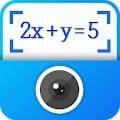# Camera Calculator – Solve Math by Take Photo APK

You just need to take photo for your math homework with Camera Calculator – Smart Calc and you can get a solution instantly. Snap Math is a multifunctional calculator which contains Basic Calculator, Scientific Calculator, Equations Calculator and BMI. With this all-in-one calculator, you can solve all the complex math problems in an easy way.

DOWNLOAD Math Camera Calculator – Smart Math Calculator, Solve Math Problems by take photo right now and try it for FREE! We are an experience team who has already developed a Smart Calculator – Solve Math Problems by Camera. And we are devoted to developing a better calculator app to satisfy all the users.

With Math Photo – Math Problem Solver by Camera, you just need to take Photo your math homework with Calculator Pro – math scanner and you can get a solution instantly. Calculator Pro is a multifunctional calculator which contains Basic Calculator, Scientific Calculator, Equations Calculator and BMI. With this all-in-one calculator, you can solve all the complex math problems in an easy way.

FEATURES of Camera Calculator – Solve Math Problem by Take Photo:

Math Calculator – Basic Calculator
The Basic calculator in this app contains add up, subtraction, multiplication and division. What’s more, it includes the percentage calculation and brackets. It’s simple designed but has complete function.

Smart Calculator – Snap Calculator
Camera calculator – Snap Calc is the most creative function of this calculator. Unlike the old calculator, you don’t need to input all the exercises one by one but take a picture and then you can get the solutions at once.

Calculator pro – Scientific Calculator
Slide up from the bottom of the keyboard and the scientific calculator will be shown. This all-in-one scientific calculator contains all the scientific calculations based on Casio 570. You can do trigonometric calculation, anti-trigonometric calculation, power calculation, logarithmic calculation, square root calculation, factorial calculation, π calculation and so on.

Multi Calculator – Equation Calculator
Are you annoying by the complex equation homework? Are you still doing it by hand? Please forget this old method now. With Smart calculator – Math Photo, you just need to enter the formula and the equation calc will give you answers automatically.

Simple Interface – Function calculator
We insist that the simple is the best, especially for calculator. Camera Calculator – Take Photo to Solve Math is simple but it still keep all the beauty factors. You’ll enjoy doing calculations with our calculator.

Calculation history
In Math calculator – Snap Math, you can slide down from the top of the keyboard and your calculation history will be shown. It shows all the calculation you done before so that you can copy, delete or reuse them.

Smart Calculator is not only just a calculator, it's also an useful homework checker. Camera Calculator can help you check your math homework after you finish it. If your answer is wrong, we'll also correct it for you.

And Calculator pro is also…
– Unit Converter
– Photo calculator
– Currency Converter
– Discount Calculator
– World Time Converter
– GPA Calculator
– Basic Calculator
– Math Camera
– Math scanner
– Solve Multiple Problems
– Scientific Calculator
– Calculation history
– Equation calculator
– Floating Window
– Great design
– Vibrate and Sound
– BMI calculator
– Math Photo
– Unit Price Calculator
– Health Calculator, BMI, BMR

Smart Calculator – Math Photo, Take Photo to Solve Math is a FREE calculator app which contains basic calculator, scientific calculator, equation calculator, BMI calculator and special Camera calculator. With the math calculator function, you can snap the math problems and get answers at once when you’re doing math homework. Smart calculator will assist you with all the math problems.